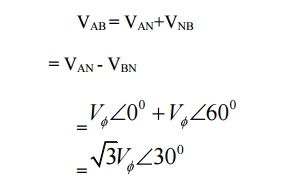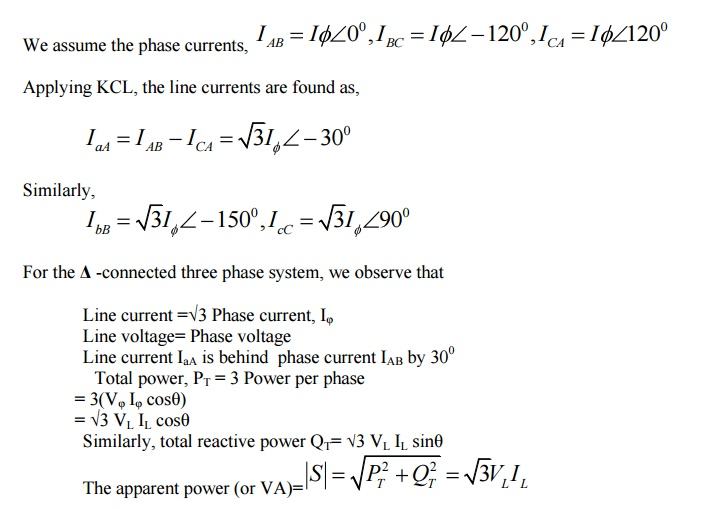Home | | Circuit Theory | Analysing Three Phase Circuits

# Analysing Three Phase Circuits

a. For household applications, we use single phase AC supply. But industries or big consumers are consuming large amount of power. b. Single phase supply is not sufficient for producing large amount of power. c. The large amount of power can be obtained from three phase AC supply.

ANALYSING THREE PHASE CIRCUITS

introduction:

a.      For household applications, we use single phase AC supply. But industries or big consumers are consuming large amount of power.

b.     Single phase supply is not sufficient for producing large amount of power.

c.      The large amount of power can be obtained from three phase AC supply.

d.     Advantages of Three phase Supply:

a.   Most of the electric power is generated and distributed in three-phase.

1.     The instantaneous power in a three-phase system can be constant.

2.     The amount of power, the three-phase system is more economical that the single-phase.

b.     In fact, the amount of wire required for a three-phase system is less than that required for an equivalent single-phase system

c.      Three phase induction motors are self starting unlike single phase induction motors.

d.     Three phase machines have better power factor and efficiency.

e.      For the same size, the capacity of a three phase machine is higher.

Phasor diagram of three phase supply:

Star Connection:Connection of Three phases supply:

1 Star connection:

a.      The terminals R, Y and B are connected together to form the star point, also called the neutral

(N).

b.The lines R, Y and B are connected to the load. If the neutral is connected to the neutral of the load.Line Voltage:

The voltage between the any two lines is called line voltage.

Phase Voltage:

The voltage between the line and neutral point is called phase voltage.

Relation between the line voltage and phase voltage:Line current:

o   The current through the line is called line current.

Phase current:

o   The current through in any phase winding is called Phase current.

Relation between the line current and phase current:

IL=IPH

i.     Delta Connection:

1)     The end of the one winding R is connected to the start of the next phase winding Y. this connection form delta or mesh connection.

Relation between the line voltage and phase voltage:

VL=VPH

Relation between the line current and phase current:ii.     Comparison of star and delta connection:

ü    Loads connected in delta dissipate three times more power than when connected in star to the same supply.

ü    For the same power, the phase currents must be the same for both delta and star connections (since power=3I p2Rp), hence the line current in the delta connected system is greater than the line current in the corresponding star-connected system.

ü    To achieve the same phase current in a star-connected system as in a delta-connected system, the line voltage in the star system is √ 3 times the line voltage in the delta system.

ü    Thus for a given power transfer, a delta system is associated with larger line currents (and thus larger conductor cross sectional area) and a star system is associated with a larger line voltage (and thus greater insulation).

b.  Three phase balanced and unbalanced voltage sources:

i.     Balanced Voltage Sources:

5)     If the voltage source have the same amplitude and frequency ω and are out of phase with each other by 120o, the voltage are said to be balanced

ii.     Unbalanced Voltage Sources:

6)     If the voltage source have the different amplitude and frequency ω and are out of phase with each other by 120o, the voltage are said to be balanced

7)     A balanced load is one in which the phase impedances are equal in magnitude and in phase

8)     An unbalanced load is one in which the phase impedances are different in magnitude and phases.

9)     Unbalanced 3 wire star connected load

10)            Unbalanced 4 wire star connected load

11)            Unbalanced 3 wire delta connected load

b.  Analysis of 3phase 3 wire with star & delta connected loads:

Consider  a  Y-connected  load.  We  will  derive  the  relationships  of  voltage,  current  and  power  for  this connection.Fig. Three phase Y-connection and phasor diagram

Assume that we are given the phase voltages (sequence ABC):We want to find the line voltages VAB, VBC and VCA.

Using KVL,

VAB = VAN+VNBNB

= VAN - VBNThis can be seen in the phasor diagram. Similarly, you can find the other line voltages as,See the phasor diagram above.

For the Y-connected three phase system, we observe that:

Line voltage =√3 Phase Voltage

Line current, IL = Phase current, Iφ

Line voltage VAB is ahead of phase voltage VAN by 300

Total power, PT = 3 Power per phaseNote: The power factor angle θ is the angle between phase voltage VAN and phase current IAN.

Consider now a -connected load. The circuit connection and phasor diagram showing the voltages and currents for the balanced circuit is shown below.Note again: The power factor angle θ is the angle between phase voltage VAB and phase current IAB.

c.       Measurements of power & power factor in 3phase circuits:ii.       Two wattmeter method

The connection and phasor diagram are shown for an assumed abc phase sequence and lagging power factor.Fig. Two-wattmeter method- connection diagram and phasor diagram

The watt-meter readings are given by,The sum of the two watt-meter readings gives the total three phase power,The power factor angle can also be found from,one of the watt-meters will give negative readings. In the laboratory, when you have made the proper watt-meter connections, you will observe that one of the watt-meters is trying to read backwards. After switching the power supply off, reverse the connection of the voltage coil or the current coil (not both). The meter will now read upscale. Assign a negative sign to this reading.

1.     The method is applicable for balanced as well as unbalanced loads.

2.     Only two wattmeter sufficient to measure total 3 phase power.

3.     If the load is balanced not only the power but power factor also can be determined. Disadvantages:

1.     Not applicable for 3 phases, 4 wire system.

2.     The sign of w1 & w2 must be identified and noted down correctly otherwise it may lead to the wrong result.

Problems

1.     The input power to a 3-phase a.c. motor is measured as 5kW. If the voltage and current to the motor are 400V and 8.6A respectively, determine the power factor of the system?

Power      P=5000W,

line voltage VL = 400 V,

line current, IL = 8.6A and

power,    P =√3 VLIL cos φ

Hence

power factor = cos φ = P √3 VLIL

= 5000 √3 (400) (8.6)

= 0.839

2.     Two wattmeters are connected to measure the input power to a balanced 3-phase load by the two-wattmeter method. If the instrument readings are 8kW and 4kW, determine (a) the total power input and (b) the load power factor.

(a)Total input power,

P=P1 +P2 =8+4=12kW

(b) tan φ =√3(P1 − P2)/(P1 + P2)

=√3 (8 – 4) / (8 + 4)

=√3 (4/12)

=√3(1/3)

= 1/ √3

Hence                   φ= tan−1 1 √3       =30◦

Power factor=      cos φ= cos 30◦ =0.866

3.     Two wattmeters connected to a 3-phase motor indicate the total power input to be 12kW. The power factor is 0.6. Determine the readings of each wattmeter.

If the two wattmeters indicate P1 and P2 respectively

 Then P1 + P2 = 12kW ---(1)

tan φ =√3(P1 − P2)/(P1 + P2)

And   power factor=0.6= cos φ.

Angle φ= cos−10.6=53.13◦ and

tan 53.13◦ =1.3333.

Hence

1.3333 =√3(P1 − P2)/12

From which,

P1 − P2          = 12(1.3333) /√3

i.e.    P1 −P2 =9.237kW          ----(2)

Adding Equations (1) and (2) gives:

2P1 = 21.237

i.e     P1 = 21.237/2

= 10.62kW Hence wattmeter 1 reads 10.62kW From Equation (1), wattmeter 2 reads

(12−10.62)=1.38kW

4.     Three loads, each of resistance 30, are connected in star to a 415 V, 3-phase supply. Determine

(a) the system phase voltage, (b) the phase current and (c) the line current.

A ‘415 V, 3-phase supply’ means that 415 V is the line voltage, VL

(a) For a star connection, VL =√3Vp Hence phase voltage, Vp = VL/√3

= 415 /√3

= 239.6 V or 240 V

correct to 3 significant figures

(b) Phase current, Ip = Vp/Rp

= 240/30

= 8 A

(c) For a star connection, Ip = IL Hence the line current, IL = 8 A

5.     Three identical coils, each of resistance 10ohm and inductance 42mH are connected (a) in star and (b) in delta to a 415V, 50 Hz, 3-phase supply. Determine the total power dissipated in each case.

(a) Star connection

Inductive reactance,

XL =2πf L =2π (50) (42×10−3) =13.19

Phase impedance,

Zp =√(R2 +XL2)

=√(102 +13.192) =16.55

Line voltage, VL =415 V

And phase voltage,

VP =VL/√3=415/√3=240 V.

Phase current,

Ip =Vp/Zp =240/16.55=14.50 A. Line current,

IL =Ip =14.50 A.

Power factor= cos φ=Rp/Zp =10/16.55 =0.6042 lagging.

Power dissipated,

P =√3 VLIL cos φ =√3 (415) (14.50)(0.6042) = 6.3kW (Alternatively,

P =3I2R =3(14.50)2(10)=6.3kW)

(b) Delta connection

VL = Vp = 415 V,

Zp = 16.55_, cos φ = 0.6042 lagging (from above). Phase current,

Ip =Vp/Zp =415/16.55=25.08A. Line current,

IL =√3Ip =√3(25.08)=43.44A.

Power dissipated,

P =√3 VLIL cos φ

=√3 (415)(43.44)(0.6042) = 18.87kW

(Alternatively,

P =3I2R

=3(25.08)2(10) =18.87 kW)

6.     A 415V, 3-phase a.c. motor has a power output of 12.75kW and operates at a power factor of 0.77 lagging and with an efficiency of 85 per cent. If the motor is delta-connected, determine (a) the power input, (b) the line current and (c) the phase current.

(a)  Efficiency=power output/power input.

Hence

(85/100)=12.750 power input from which, Power input = 12. 750 × 10085

= 15 000W or 15Kw

(b)  Power, P=√3 VLIL cos φ, hence

(c)    line current,

IL = P/ √3 (415) (0.77)

= 15 000/ √3 (415) (0.77)

= 27.10A

(d)  For a delta connection, IL =√3 Ip,

Hence

Phase current, Ip = IL/√3

= 27.10 /√3

= 15.65A

7.     A 400V, 3-phase star connected alternator supplies a delta-connected load, each phase of which has a resistance of 30_ and inductive reactance 40_. Calculate (a) the current supplied by the alternator and (b) the output power and the kVA of the alternator, neglecting losses in the line between the alternator and load.

A circuit diagram of the alternator and load is shown in Fig.

Phase current, Ip =Vp/Zp

Vp =VL for a delta connection,

Hence Vp =400V.

Phase impedance,

Zp =√ (R2+XL2)

=√ (302 +402) =50Figure

Hence Ip =Vp/Zp =400/50=8A.

For a delta-connection,

Line current, IL =√3 Ip =√3 (8) =13.86 A.

Hence 13.86A is the current supplied by the alternator.

(b) Alternator output power is equal to the power Dissipated by the load

I.e. P =√3 VLIL cos φ, Where cos φ = Rp/Zp = 30/50 = 0.6.

Hence P =√3 (400) (13.86) (0.6) = 5.76kW.

Alternator output kVA,

S =√3 VLIL =√3 (400) (13.86)

9.60 kVA.

Study Material, Lecturing Notes, Assignment, Reference, Wiki description explanation, brief detail
Electrical and electronics : Circuit Theory : Analysing Three Phase Circuits : Analysing Three Phase Circuits |